# Quiz Mathematics

Last Updated:

## Improve your knowledge with our Mathematics Quizzes

Mathematics is a fundamental discipline that plays an essential role in many areas of knowledge. Whether you’re a numbers enthusiast or just curious, our math quizzes will help you test your knowledge of mathematical concepts, famous theorems, formulae and problems.

Do you know the basic principles of arithmetic, algebra and geometry? Can you solve complex equations and logic problems? Do you know the great mathematicians of history and their contributions? Can you understand and apply the concepts of probability and statistics?

Our math quizzes will help you discover new concepts and strengthen your understanding of mathematics. Math quizzes are a fun way to expand your knowledge and test your math skills. So, if you’re passionate about math or just curious, come and test your knowledge with our math quizzes.

sciences

/ 10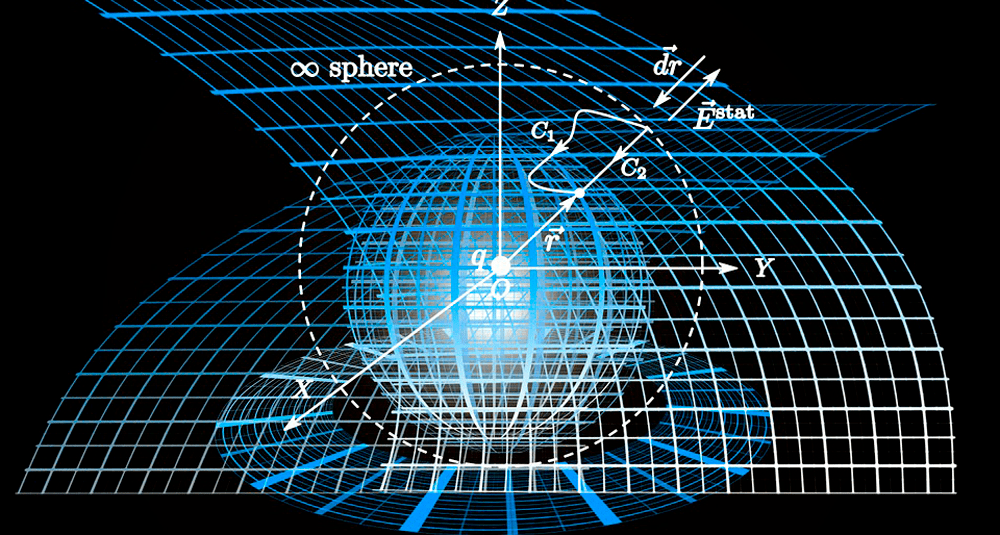How many vertices does a hexagon have?

16

210A hexagon is a polygon with six vertices and six sides. A hexagon can be regular or irregular.

Next questionA hexagon is a polygon with six vertices and six sides. A hexagon can be regular or irregular.

Next question

### 0 / 10

New Quiz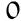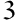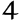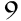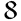Players